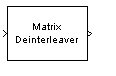Main Content

# Matrix Deinterleaver

Permute input symbols by filling matrix by columns and emptying it by rows

## Library

Block sublibrary of Interleaving

•## Description

The Matrix Deinterleaver block performs block deinterleaving by filling a matrix with the input symbols column by column and then sending the matrix contents to the output port row by row. The Number of rows and Number of columns parameters are the dimensions of the matrix that the block uses internally for its computations.

This block accepts a column vector input signal. The length of the input vector must be Number of rows times Number of columns.

The block accepts the following data types: `int8`, `uint8`, `int16`, `uint16`, `int32`, `uint32`, `boolean`, `single`, `double`, and fixed-point. The output signal inherits its data type from the input signal.

## Parameters

Number of rows

The number of rows in the matrix that the block uses for its computations.

Number of columns

The number of columns in the matrix that the block uses for its computations.

## Examples

If the Number of rows and Number of columns parameters are 2 and 3, respectively, then the deinterleaver uses a 2-by-3 matrix for its internal computations. Given an input signal of `[1; 2; 3; 4; 5; 6]`, the block produces an output of `[1; 3; 5; 2; 4; 6]`.

## Pair Block

Matrix Interleaver

## See Also

### Blocks

Introduced before R2006a

## Support

#### Bridging Wireless Communications Design and Testing with MATLAB

Download white paper